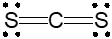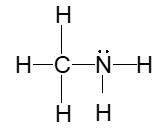Start typing, then use the up and down arrows to select an option from the list.## General Chemistry

Learn the toughest concepts covered in Chemistry with step-by-step video tutorials and practice problems by world-class tutors

12. Molecular Shapes & Valence Bond Theory

# Electron Geometry

The simplest system for geometrical shapes that focuses on the number of electron groups around the central atom.

Electron Geometry
1
concept

## Electron Geometry Concept 13m
Play a video:
Hey everyone. So when we say electron geometry realize that it represents the simplest system for geometrical shapes that focuses on the number of electron groups around the central element. And when it comes to the electron geometry, we're gonna treat lone pairs and surrounding elements as the same. So if we take a look here at this chart, we're breaking things down in terms of number of electron groups, the orbital shapes where we just give certain examples that fit this particular row, the electron geometry name and then a memory told to help us to remember that electron geometry name. So here we're gonna start out with the first one. And let's say that our molecule has two electron groups. Here we have carbon dioxide as as an example, carbon is a central element. It has two surrounding elements. So that's why it has two electron groups. The geometry here the electron geometry would be called linear. And a good way to remember linear. Is that recall that there are two points in a straight line. Okay, so line for linear and two points because there's two electron groups. So again, we have carbon in the center and we have two electron groups. These two oxygen's For the next one. We have three electron groups. And remember these electronic groups could either be three surrounding elements Or there could be two surrounding elements and one lone pair. Remember we're treating lone pairs and surrounding elements as the same in this case. The electron geometry name would be tribunal plainer. Now the beauty of chemistry is that we can pronounce things differently, but all that really matters is that you get the spelling correct? Okay, so you might hear trickle planner, Trigano Planner, Tribunal planner Trigano Planner. None of that matters. Just make sure you're spelling it correctly and how to remember this. Well we're gonna say we have three electron groups and it starts with tri tri meaning three. Next we have four electron groups. And remember that could just be different situations where they're all surrounding alliance or their surrounding elements with a mixture of lone pairs as long as it adds up to four electron groups. It fits in this row here. The electron geometry name would be tetra hydro tetra means four for electron groups. Now the next two Let's look at the next two We have five electron groups for the next one. So the central element has five electron groups around it. Which could be all surrounding elements or a combination of surrounding elements and lone pairs. Right so here we have three surrounding elements. Two long pairs still that's up to five. Here we have two surrounding elements in three lone pairs. Now the name for this one. This one's a bit longer. This is tribunal or Trigon. All by pyramidal. Now jules. How am I supposed to remember this name? Well we know that it has five electron groups and we're gonna say your try means three by here means to three plus two will give me five. Alright, finally we have six electron groups. So again that could be a combination of all surrounding elements That add up to six or some combination of surrounding elements and long pairs. This one, its name is federal. This one is gonna require a little bit more imagination in terms of remembering it Octa you might say hey jules, octo means eight. But here we're talking about six electron groups. Well we can play on this idea of optimizing eight. So Doctor octopus. So an octopus has eight tentacles right or eight legs And it has two months. So eight tentacles -2, -2 gives me six six electron groups. So remember if you have six electron groups, your electron geometry is octahedron. So just remember some of these memory tools. If it's hard for you to remember a particular electron geometry name and you'll be able to get through this. Remember in the case of electron geometry, lone pairs on the central element and surrounding elements are treated as the same

The electron geometry of a compound treats surrounding elements and lone pairs on the central element as the same.

2
example

## Electron Geometry Example 148s
Play a video:
determine the electron geometry for the hydrogen sulfide molecule H two S. So if we take a look at hydrogen sulfide or yeah, sulfide molecule, we're going to see here that it is a central element, sulfur connected to how many electron groups it's connected to 1234 electron groups. Remember electron groups represent lone pairs on the central element as well as surrounding elements directly connected to the central element. So we have four electron groups and remember four is connected to tetra because it's connected to tetra. This electron geometry B Catron federal. So hydrogen sulfide has a tetra hydro electron geometry.
3
Problem

Determine the electron geometry for the carbon disulfide molecule, CS2A. Linear

B. Bent

C. Trigonal planar

D. Tetrahedral

4
example

## Electron Geometry Example 21m
Play a video:
recall there are many possible lewis dot structures that exist. But there are rules to draw the best structure. So for this example, it says determined the electron geometry for the following molecule CH two. Oh, this is also called formaldehyde. Um So if we take a look here, we place the least electro negative element in the center. Now that would be hydrogen. But remember we have another rule when it comes to hydrogen, it can never go in the center. So as a result it's carbon that has to go in the center. Carbon is in group four a. So it has four valence electrons. And Remember Hydrogen Czar Group one and they can only form single bonds. Mhm Carbon itself has to make four bonds. It's already making two bonds and eat two more. The only element that's left is oxygen. So carbon would have to form a double bond to that oxygen. Carbon, oxygen and group's success. What has six valence electrons and here we're showing all of them. So this would represent the structure or molecular shape of formaldehyde. Now, if we take a look, we'd say that the carbon is connected to how many electron groups It's connected to one, 2. 3 electron groups. Remember three. We say try equals three. Because we're saying try that would mean that the electronic geometry is tribunal planer. So this would be the electronic jammed your molecular shape of the formaldehyde molecule
5
Problem

Determine the number of electron groups for the following cation:AsBr2+

A. 2

B. 3

C. 4

D. 1

6
Problem

Draw and determine the electron geometry for the following molecule:TeBr4

7
Problem

Determine the electron geometry of the nitrogen atom within methylamine, CH3NH2.A. Linear

B. Trigonal Pyramidal

C. Tetrahedral

D. Trigonal Planar